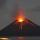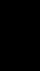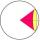# Cone + area - math problems

#### Number of problems found: 70

• VolcanoThe crater of a volcano is approximately in the shape of a cone of a base 3.1416 square miles. The crater's depth is 1500 ft. How many cubic yards of earth would be required to fill this cavity?
• Cone A2VThe surface of the cone in the plane is a circular arc with central angle of 126° and area 415 cm2. Calculate the volume of a cone.
• How manyHow many m2 of copper sheet is needed to replace the roof of a conical tower with a diameter of 13 meters and a height of 24 meters if we count 8% of the material for bending and waste?
• Frustrum - volume, areaCalculate the surface and volume of the truncated cone, the radius of the smaller figure is 4 cm, the height of the cone is 4 cm and the side of the truncated cone is 5 cm.
• Cone and the ratioThe rotational cone has a height 43 cm, and the ratio of the base surface to lateral surface is 5: 7. Calculate the surface of the base and the lateral surface.
• A concrete pedestalA concrete pedestal has a shape of a right circular cone having a height of 2.5 feet. The diameter of the upper and lower bases are 3 feet and 5 feet, respectively. Determine the lateral surface area, total surface area, and the volume of the pedestal.
• Angle of deviationThe surface of the rotating cone is 30 cm2 (with circle base), its surface area is 20 cm2. Calculate the deviation of the side of this cone from the plane of the base.
• Rotary coneThe volume of the rotation of the cone is 472 cm3, and the angle between the side of the cone and the base angle is 70°. Calculate the lateral surface area of this cone.The cone-shaped lampshade has a diameter of 30 cm and a height of 10 cm. How many cm2 of material will we need when we 10% is waste?
• Cone sideCalculate the volume and area of the cone whose height is 10 cm and the axial section of the cone has an angle of 30 degrees between height and the cone side.
• Cone - from volume surface areaThe volume of the rotating cone is 1,018.87 dm3, and its height is 120 cm. What is the surface area of the cone?
• Rotary bodiesThe rotating cone and the rotary cylinder have the same volume of 180 cm3 and the same height v = 15 cm. Which of these two bodies has a larger surface area?
• SphereIntersect between plane and a sphere is a circle with a radius of 60 mm. Cone whose base is this circle and whose apex is at the center of the sphere has a height of 34 mm. Calculate the surface area and volume of a sphere.
• ConeCalculate volume and surface area of ​​the cone with a diameter of the base d=15 cm and side of the cone with the base has angle 52°.
• CalculateCalculate the cone's surface and volume that results from the rotation of the right triangle ABC with the squares 6 cm and 9 cm long around the shorter squeegee.
• Lateral surface areaThe ratio of the area of the base of the rotary cone to its lateral surface area is 3: 5. Calculate the surface and volume of the cone, if its height v = 4 cm.
• Castle towerThe castle tower has a cone-shaped roof with a diameter of 10 meters and a height of 8 meters. Calculate how much m² of coverage is needed to cover it if we add one-third to the overlap.
• Conical areaA right angled triangle has sides a=12 and b=19 in right angle. The hypotenuse is c. If the triangle rotates on the c side as axis, find the volume and surface area of conical area created by this rotation.
• TowerHow many m2 of the copper plate should be replaced on the roof of the tower conical shape with diameter 24 m, and the angle at the axial section's vertex is 144°?
• The diagram 2The diagram shows a cone with slant height 10.5cm. If the curved surface area of the cone is 115.5 cm2. Calculate correct to 3 significant figures: *Base Radius *Height *Volume of the cone

Do you have an exciting math question or word problem that you can't solve? Ask a question or post a math problem, and we can try to solve it.

We will send a solution to your e-mail address. Solved examples are also published here. Please enter the e-mail correctly and check whether you don't have a full mailbox.

Cone Problems. Area - math problems.